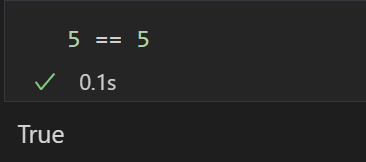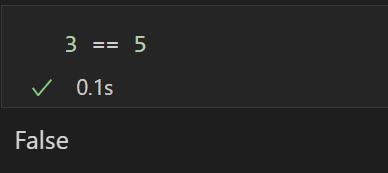A lot of elements of mathematical logic are used in programming. These can be tricky to understand, especially when wrapped inside of the syntax of a programming language.

A good example are lambda expressions. These are commonly used in various scenarios, yet they can look somewhat cryptic the first few times we come across them. In this post, we will be defining lambda expressions, looking at the two most common contexts where they are used, and giving some examples.

## What are lambda expressions?

A lambda expression is defined in computer programming as an anonymous function, which are functions without a name.

Most people will be familiar with the following mathematical syntax to define a function: `f(x) = x*x` or `square(x) = x*x`. Both the function called “f” and the function called “square” take the value of x and compute the square of that number. Both functions perform the exact same computation, so both functions will have the same corresponding lambda expression. The only difference is their name, hence anonymising them yields the same result.

The exact mathematical definition of functions doesn't really matter here. It suffices to understand that a function (and hence a lambda expression in computer programming) is an object that, given an input, produces an output. Here, given x (the input), x*x will be computed (the output). So if the input is 2, the output will be 4.

Now that we know that lambda expressions are anonymous functions and what that means, let's see how these are used in programming.

## Lambda Expressions in C#

Lambda expressions are used in programming to make code more easily readable. They are useful when the function is short and simple, and defining it separately and giving it a descriptive name is not necessary. Lambdas are syntactically light, yet they can take a while to get used to. Note that each programming language has a different syntax for them. In this post, we will be giving examples in C# only.

In C#, we can write a lambda expression that computes the square of its input with this syntax:

``````x => x*x
``````

There are two kinds of lambda expressions:

• Expression lambdas are lambda expressions where the body (the right hand side) is an expression, such as x*x in the example we saw earlier.
• Statement lambdas are lambda expressions whose bodies are blocks of code.

Let's look at the two most common situations where you'll use lambda expressions – in LINQ expressions and to define delegates.

## Lambda Expressions in LINQ

LINQ (Language Integrated Query) is a set of technologies used in C# to query data of different types. LINQ can use query expressions, lambda expressions, extension methods, anonymous types, and various library functionalities.

There are two possible ways to query objects using LINQ – using query syntax or using method syntax. Both yield the same result with different syntaxes, as query expressions are converted into a method call and a lambda by the .NET common language runtime (CLR). It is useful to explore both of them when learning about LINQ, but for the purposes of this blog post, we will only work with lambda expressions.

Let's look at some examples of lambda expressions used in LINQ.

1. Expression Lambdas
``````var numbers = new int[] {2, 7, 5, 9, 1, 5, 3, 0, 7, 6};

var count = numbers.Count( x => x==5 );
Console.WriteLine(count);
``````

This code returns the number of times that 5 is present in the list “numbers”. The LINQ query used here is `Count`, which takes a filter expression and counts the items that satisfy the expression. How does `Count` determine which items to include in the count? Using the lambda expression `x => x==5`.

You'll notice this lambda expression is a little different from the examples of functions we gave earlier. In this case, the output won't be a number, but rather a Boolean. This expression checks whether the given input x is equal to 5. If it is, a value of True will be returned, otherwise False will be the output. This is called a predicate, a statement that, depending on the values of its variables, will be true or false.So `numbers.Where( x => x==5 )`, is saying “query the list “numbers” when the lambda expression (x => x==5) evaluates to True”.

1. Statement Lambdas

I tend to find expression lambdas (where the body is an expression, like x == 5 here) easier to understand than statement lambdas (where the body is a block of code, a sequence of statements enclosed in braces). Let's look at a second example.

``````List<int> numbers = new List<int> {2, 7, 5, 9, 1, 5, 3, 0, 7, 6};

var count = numbers.Count( x =>
{
return x == 5;
});

Console.WriteLine(count);
``````

This example is equivalent to the example we had before, this time using a statement lambda. This time, the lambda expression used inside of the count is

``````X => { return x == 5; }
``````

Using a statement lambda here is unnecessary, but it is enough to show the syntax. `return x==5` will return a boolean, just like the expression in our earlier lambda. Then the `Count` will count the number of items satisfying the expression.

## Lambda Expressions in Delegates

So far, we have seen how lambda expressions are used in LINQ, but they are not constrained to this. They can also be used to create delegates.

Let's look at two simple examples, one using expression lambdas and one using statement lambdas.

1. Expression Lambdas

When using expression lambdas, a delegate can be created using the `Func<T>` class.

``````Func<int, int> squareDelegate = x => x*x;
Console.WriteLine(sumDelegate(2));
``````

`squareDelegate` takes an integer as an input, and outputs a value of type integer as well. The lambda expression is the same we used for our very first set of examples. The value of the input x will be used to compute the output in the expression x*x.

1. Statement Lambdas

A delegate can be created in the same way using a statement lambda.

``````Func<int, int> exampleDelegate = (x =>
{
return x*x;
});

Console.WriteLine(exampleDelegate(2));
``````

Here, the expression side of the lambda is a statement, a block of code enclosed in braces. This syntax can look more involved, but the result is the same. The value of x passed into the delegate when called, and will be used to calculate the square of x.

## Conclusion

In this post, we have seen the definition and different types of lambda expressions, and how they can be described as anonymous functions. We have seen that their use is very common in LINQ queries and in delegates, alongside some simple examples.

### Elisenda Gascon

#### Apprentice Engineer IIElisenda was an Apprentice Engineer from 2021 to 2023 at endjin after graduating with a mathematics degree from UCL. Her passion for problem solving drove her to pursue a career in software engineering.

During her time at endjin, she helped clients by delivering data analytics solutions, using tools such as Azure Synapse, Databricks notebooks, and Power BI. Through this, she also gained experience as a consultant by delivering presentations, running customer workshops, and managing stakeholders.

Through her contributions to internal projects, she gaines experience in web development in ASP.NET, contributed to the documentation of the Z3.Linq library, and formed part of a team to develop a report to explore global trends in wealth & health.

During her training as a software engineer, Elisenda wrote a number of blog posts on a wide range of topics, such as DAX, debugging NuGet packages, and dependency injection. She has also become a Microsoft certified Power BI analyst and obtained the Green Software for Practitioners certification from the Linux Foundation.